### Ecological Archives E081-027-A5

Frederick R. Adler, and Julio Mosquera. 2000. Is space necessary? Interference competition and limits to biodiversity. Ecology 81:3226-3232.

Appendix E: Results with non-spatial model.

We assume that the function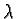is decreasing and that there exists a value smax that solves the equation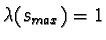(the species with the largest value of s that could replace itself in the absence of competition). We also assume that the second derivative of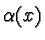is negative for x > 0.

First, we show that the non-spatial model supports a continuum of species if the competition function is discontinuous with the form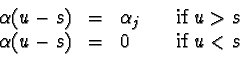All members of a coalition must satisfy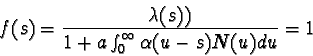where the integral is taken over all members of the coalition. We show that the coalition consists of all species with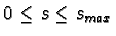. The condition that f(s)=1 can be rewritten as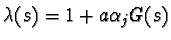where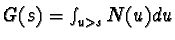. Differentiating, we find that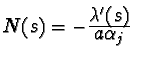. This solution is the uninvadible coalition.

Second, suppose that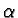andare both analytic. The integral in the condition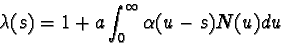is a convolution (as in Appendix B), hence both sides are analytic. Therefore, if the coalition includes an interval, both sides must be equal over their entire domains. However,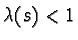if s > smax, while the right hand side must always exceed 1. Therefore, the coalition can include no intervals.

As in Appendix C, any uninvadible single species must be at the minimum value of s (this requires that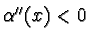for x > 0). Similarly, it can be shown that one member of any two species coalition must have s=0. Suppose the coalition consists of N0 seeds of the species with s=0 and N1 seeds of a species with s=z1. For convenience, and without loss of generality, we scale the parameter a to be equal to 1. Necessary conditions for dynamic and evolutionary stability are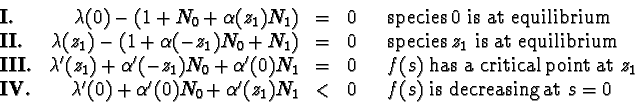Suppose that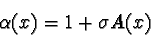(6)

where A(x) is odd and A'(0)=1Then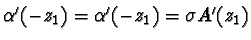. The first three conditions can be solved for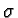, N0 and N1, and the result substituted into inequality IV, which becomes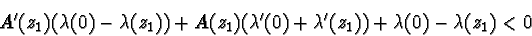If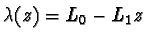, this condition simplifies to

L1 (A'(z1) z1 - 2 A(z1) + z1) < 0 .

This condition on the function A exactly matches the condition on the function b0 in equation 4 from Appendix D. Therefore, the conditions for a existence of a two species coalition are identical to those in the spatially-structured model, which implies also that N1 > N0.

If we Taylor expand the function(assuming it is differentiable) around x=0, the first two terms are given by equation 6. Similarly, if we Taylor expand the function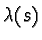around s=0, the first two terms are given by the linear form. Therefore, near s=0 where the bifurcation takes place, condition 4 determines whether a two species coalition will exist.

The necessary occurrence of the bifurcation from one species to two might seem in conflict with some results in Rees and Westoby (1997). With their first model, which takes the form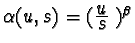when written in terms of seed size, coexistence is impossible. However, this function is not a function of u-s, has a slope that does not increase continuously as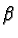increases, and has the wrong concavity when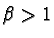.

More importantly, this competitiveness function can be written as a ratio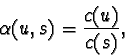where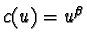in this case. The expression for the per seed reproduction f(s) can be rewritten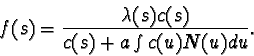All species experience the same value of the integral which can thought of as a surrogate for resource depletion (Adler, 1990). The species that can persist with the maximum level of resource depletion (which in this case maximizes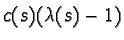) is uninvadible. The fact thatcan be written as a ratio, not that it is unbounded (as argued by Rees and Westoby (1997)), leads to the lack of coexistence.

Literature Cited

Adler, F. R. 1990. Coexistence of two types on a single resource in discrete time. Journal of Mathematical Biology 28: 695-713.
Rees, M., and M. Westoby. 1997. Game-theoretical evolution of seed mass in multi-species ecological models. Oikos 78: 116-126.

[Back to E081-027]